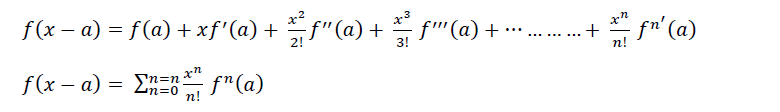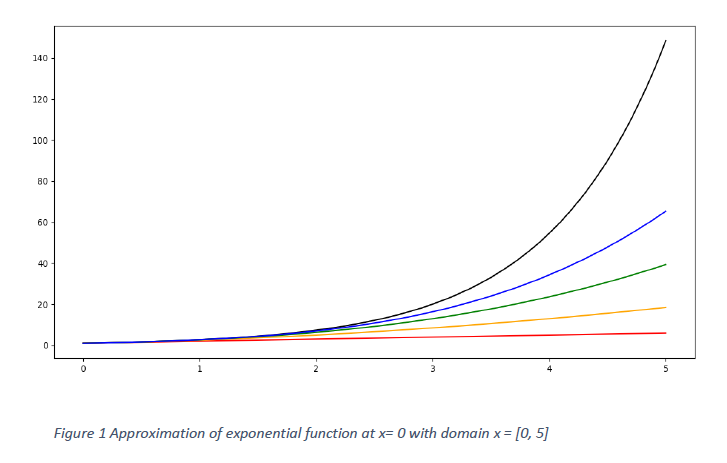Search
•Anand Zambare

# Taylor's Series : My favourite series of all time

Updated: Jul 2, 2021

"Approximations are not always bad, they are accurate until you can resolve between the reality and approximation. Taylor's Series: an approximation with reality"

### Introduction :

Hello, Mech n Flow family!! I hope you enjoyed the last article of introduction to computational fluid dynamics and you are excited to go ahead into the sea of CFD. As we discussed at the end of the last article the topic for this article is Taylor’s Series, which is the second article of the series on CFD, in case if you have missed the first article you can find it here.Taylor's series

Mathematics is the backbone of CFD, we will be discussing the full foundation of mathematics required to build the tall building of CFD. The most fundamental thing required to start with is Taylor’s Series. Now here it is expected that the reader should be having knowledge of derivatives, functions and basic XII (10 + 2) mathematics.

Before going ahead into the article we would like to ask a question to readers, why do we use co-ordinate system to solve the problems? Let us know in comments.

### Domain of the problems :

Consider a pipe flowing with water, the pipe is full of water. The 2-D domain for this case will be as shown in the image below. The domain of the problem is marked using a specific co-ordinate system which is basically orthogonal in nature. Different properties in this domain are mapped using different functions and this we need to take into account while moving ahead in the section of the Taylor’s Series. We are considering the rectangular co-ordinate system which has origin in the corner. Such representation of domain can represent not only pipe flow but also many 2-D scenarios like flow over a flat plate.### Role of Functions :

Again we are considering the situations defined earlier i.e. of pipe flow. The rectangular geometry has four boundaries and it forms our domain. As we have seen that solving a flow problem is nothing but solving for unknown properties of flow like Temperature (T), Velocity (V) and so on. So these properties are nothing but defined as some functions in the domain like T(x, y, t) and V(x, y, t). These functions can be anything, figuring out them is our task. It is important to visualize the functions before we start looking at Taylor’s Series.

### Taylor's Series :

Now that we are familiar with how functions can play the important role we can look at Taylor’s series. Let us define Taylor’s Series first,

“It is the tool using which we can express any of the given function in terms of polynomial function. Higher is the order of polynomial better will be the accuracy of approximation.”

Let’s look at it in mathematical sense, let f(x) be any function and we want to express it as a series of polynomial function about a point x-a, then we can writeFirst equation represents the Taylor’s series for a function f(x) about a point a in the given domain. It is possible to write it using notations to make it little simple and next equation is representation of that only. There exists a special case of a = 0 and such special case reduces the Taylor’s series to McLaurin series which can be given as follows in equationNow we have just got introduced to very beautiful series called as Taylor’s Series. Its time to look at the few of the examples related to Taylor’s series and in the end we will figure out the role of Taylor’s Series in CFD. For first example let us consider f(x) as exponential of x. (Consider about origin).

### Taylor's Series Approximation for exponential functions :

Consider the definition of series with a = 0, because we are expanding the series about origin. Find the derivatives and respective values as in the table below.This is the approximate polynomial of the exponential function about x = 0. Now to approximate the exponential function correctly we have to add higher degree of polynomial. So if we consider only first two term then our polynomial will be linear, if we consider first three terms then the polynomial will be quadratic, then we can make it cubic and so on. Let’s look at it graphically.Figure 1 and figure 2 are representing the exponential function in domain x = [0, 5] and x = [0, 3] respectively. In the figures the exact exponential function is represented by black color while all other colours are approximation of exponential functions. Let’s see what each color is representing.

Red color – The linear function approximation which is nothing but first two terms in Taylor’s series

Yellow color – The quadratic polynomial approximation which will be first three terms in Taylor’s series

Green color – The cubic polynomial approximation which will be first four terms in Taylor’s series

Blue color – The fourth order polynomial approximation which will be first four terms in Taylor’s series

It can be clearly seen that the higher the power of approximation the better the accuracy. The figures below will show the approximation of sine function using Taylor’s series and now I believe you can understand that easily. This is about the point x = 0, as we increase the degree of polynomial we are getting better.### Application of Taylor’s series in CFD:

As we all know that differentiation and integration are the operations which a computer can’t understand. So we use numerical techniques to solve such problems in computers and here is the biggest application of Taylor’s series.

Also Taylor’s series plays an important role in Finite Difference Method where we use it again to approximate derivatives. Let’s look at it and check how we can use it.

Let’s say we want to approximate the first derivative at a point, for that let’s consider the simple case of point about zero so we can write f(x) assince we want first derivative we can consider the series up-to that point only.Now consider the series for 𝑥+Δ𝑥 point where Δ𝑥 is known to usSo we have equation (1) and (2) with us, if we subtract equation two from equation one we directly getand by rearranging this we can writeSo here we are, now we can approximate the derivative of a function at x = 0, using the Taylor’s series. So by this method we can approximate second derivative as well higher derivatives, this sort of approach is used in Finite Difference Method (FDM).

### History :

The famous English Mathematician Brook Taylor got the recognition in the world after his discovery of Taylor’s series and his research in the mathematics. He was a son of MP of Kent and was from sufficiently wealthy family. He was selected as a fellow of Royal society in 1712 and he was in committee which reviewed the work of calculus done by Sir Isaac Newton and Leibniz. His work lead to completely different branch of mathematics called “Calculus of Finite Differences”.

In the beginning it was Greek mathematician Zeno who had thought of writing an infinite sum as a finite sum, but with some argument he decided to give up his idea, this was known as Zeno's Paradox at that time. Aristotle came up with some solution to Zeno's paradox but he couldn't give the perfect mathematical arguments. It was Archimedes with some good mathematical support came and solve the Zeno's paradox.

With some many mathematicians working for many years it is believed that Indian mathematicians were first to solve this paradox of infinite sum but there are hardly any proofs of that, it is said that they were able to obtain the series for sine, cosine and tangent function in 14th century i.e. more than 300 years before Brook Taylor.

### Summary:

• Taylor's Series is the tool using which we can express any of the given function in terms of polynomial function.

• Higher the degree of polynomial better will be the approximation.

• Taylor's series plays an important role in CFD as we can use it to approximate the derivatives of the function at a point in terms of function values at that point and near by points.

If this blog has made you curious about Taylor's series and you want to understand the more mathematics involved in it, please check the following YouTube video. 3Blue1Brown Series S2 E11. (All Credits of following YouTube Video to 3Blue1Brown).

The Process of sowing the seeds has started, let's keep our efforts to the best to grow the giant tree of Fluid dynamics. In the next blog we are going to discuss about the Finite Difference Method (FDM) and few problems also. Join us to increase the slope of your learning curve.